LOGARITHMIC DIFFERENTIATION

The following problems illustrate the process of logarithmic differentiation. It is a means of differentiating algebraically complicated functions or functions for which the ordinary rules of differentiation do not apply. For example, in the problems that follow, you will be asked to differentiate expressions where a variable is raised to a variable power. An example and two COMMON INCORRECT SOLUTIONS are :
1.)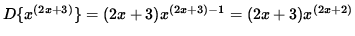and
2.).

BOTH OF THESE SOLUTIONS ARE WRONG because the ordinary rules of differentiation do not apply. Logarithmic differentiation will provide a way to differentiate a function of this type. It requires deft algebra skills and careful use of the following unpopular, but well-known, properties of logarithms. Though the following properties and methods are true for a logarithm of any base, only the natural logarithm (base e, where e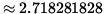),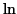, will be used in this problem set.

PROPERTIES OF THE NATURAL LOGARITHM

1..

2..

3..

4..

5..

6..

AVOID THE FOLLOWING LIST OF COMMON MISTAKES

1..

2..

3..

4.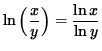.

5.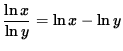.

The following problems range in difficulty from average to challenging.

• PROBLEM 1 : Differentiate y = xx .

• PROBLEM 2 : Differentiate y = x(ex) .

• PROBLEM 3 : Differentiate y = (3x2+5)1/x

• PROBLEM 4 : Differentiate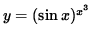.

• PROBLEM 5 : Differentiate.

• PROBLEM 6 : Differentiate.

• PROBLEM 7 : Differentiate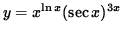.

• PROBLEM 8 : Differentiate.

• PROBLEM 9 : Differentiate.

• PROBLEM 10 : Consider the function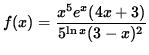. Find an equation of the line tangent to the graph of f at x=1 .

• PROBLEM 11 : Consider the function. Determine the slope of the line perpendicular to the graph of f at x=1 .

• PROBLEM 12 : Differentiate